### THE CREATION OF PLANETARY MAGNETIC FIELDS

D. Russell Humphreys*

Creation Research Society Quarterly

Volume 21, Number 3 (December 1984)

Received 3 January 1984; Revised 14 August 1984

 [The CRS acknowledges the work of Doug Sharp in converting this article into html format. For other work by Doug, you are invited to visit the Web site below.] The Revolution Against Evolution

*D. Russell Humphreys has a Ph.D. in physics and is a physicist at
Sandia National Laboratories, Albuquerque, NM 87185.

 NOTE: In this paper, Dr. Humphreys makes predictions for the strengths of the magnetic fields for Uranus and Neptune, well before these magnetic fields were measured by the Voyager spacecraft. His predictions were "right on," whereas the predictions of evolutionists were not.

Abstract

God could have started magnetic fields in the solar system in a very simple way: by creating the original atoms of the planets with many of their nuclear spins pointing in the same direction. The small magnetic fields of so many atomic nuclei add up to fields large enough to account for the magnetism of the planets. Within seconds after creation, ordinary physical events would convert the alignment of nuclei into a large electric current circulating within each planet, maintaining the magnetic field. The currents and fields would decay steadily over thousands of years, as Barnes has pointed out. The present magnetic field strengths of the Earth, Sun, Moon, and planets agree very well with the values produced by this theory and a 6000-year age for the solar system. This theory is consistent with all the known data and explains many facts which have puzzled evolutionists.

 Introduction The Earth's magnetic field is what makes compass needles point north. In an earlier paper1 I showed that God could have started the Earth's field in a very simple way, by using the magnetic fields of spinning atomic nuclei (Figure 1). He could have created many of the Earth's original atomic nuclei with their spins pointing in a particular direction. The small magnetic fields of so many nuclei would add up to a field large enough to account for the Earth's magnetism.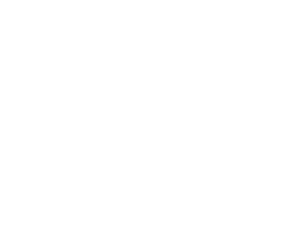Immediately after their creation, the atoms would begin to collide due to normal thermal motions. Within seconds these collisions would knock the nuclei out of their original alignment into a more random order. But the ordinary laws of electricity and magnetism would maintain the magnetic field by starting up a large electric current - billions of amperes - in the Earth's conductive interior. The process is shown in Figure 2. Figure 1. Magnetic field of an atomic nucleus. Atoms of many elements, such as hydrogen, have spinning nuclei. Such a nucleus has a small magnetic field, like that of a small bar magnet lined up with the spin axis.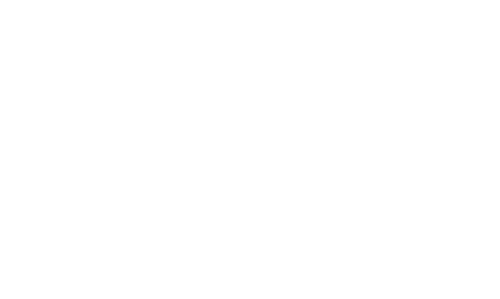Figure 2. The change from aligned nuclear spins to a circulating current in a planet. (a) God creates atomic nuclei with aligned spins, producing a magnetic field around the planet. (b) Thermal collisions of atoms begin to disorient the spins, starting up an electric current in the interior which maintains the field. (c) A few seconds after creation, the nuclei are completely disoriented. The current is fully established, and the magnetic field is as strong as before.

The electrical resistance of the interior would then cause the current and field to decay steadily over thousands of years down to the size they are today. The field would decrease exponentially, that is, by a fixed percentage per unit time (Figure 3) . (Since readers of this Quarterly come from very diverse areas of science, I am italicizing and explaining the more technical terms).

Dr. Thomas Barnes2 has used 130 years of published magnetic field observations to show that the earth's field indeed appears to be decaying exponentially at about five percent per century. He also showed that that decay rate corresponds to a reasonable value of electrical conductivity in the Earth's core.

In my article I calculated on the basis of the nuclear magnetism hypothesis that the Earth's field at creation was about eighteen times stronger than it is now. This value agrees to within five percent with the value we get by extrapolating the field's present decay rate 6000 years into the past, well within the experimental error.

A magnetic field generated and decaying in this way would have about the same shape as the field of a bar magnet. Such a field has only two poles (north and south), so physical scientists call it a dipole field. Most of the Earth's field today is dipolar.

So the nuclear magnetism idea, the short Biblical time scale. and an exponential decay all fit the main features of the Earth's field rather well. After writing the first article, I began to wonder if God made the magnetic fields of the Sun, Moon, and planets in the same way, that is, by creating the nuclei with their spins lined up.

Fortunately, the last decade of planetary exploration has produced much data against which to check the theory. The next three sections outline the theory. For details and references, see my previous article. In sections 5-9 I apply the theory to the various solar system bodies and compare the results to the known magnetic field data.

As well as I can tell, all of the data fall within the bounds of the theory. I hope that you, the reader, will find this as exciting as I do.

Water: The Raw Material of Creation

The strength of a dipole field's source is called its magnetic moment. It is proportional to the amount of current circulating in the source and the area encircled by the current. The dipole magnetic moment of the earth today is 7.9 x 1022 joules per Tesla (I J/T = 1 Ampere-meter2 = 1000 Gauss-cm3).3,4

To calculate the magnetic moment of a planet at creation, we must know the original material. In the previous article I presented Scriptural evidence that God originally created the Earth as a sphere of pure water. One of the Scriptures is the last part of 2 Peter 3:5 (NASB): ". . . and the earth was formed out of water and by water." Shortly after that, God must have transformed much of the water into other matter, such as iron, silicon, minerals, and rock.

I know of no explicit Scripture which says that God created the heavenly bodies in the same way He did the Earth. But there is a hint, perhaps. The Hebrew word translated "heavens" in Genesis 1 consists of two other Hebrew words which mean "there, waters."5 Let us assume that God created the Sun, Moon, and planets as water, which He then transformed.

Lining Up Nuclei

The next thing we need to know is bow much magnetic moment water can have. How magnetic moments in a water molecule line up under normal circumstances, and how God may have aligned them at creation are discussed in this section.

The magnetic moments of the 10 electrons in a water molecule cancel themselves out, so that their total contribution is zero. The magnetic moment of the oxygen nucleus is similarly zero. But the two hydrogen nuclei (protons) in the molecule each have a magnetic moment of 1.41 X 10-26 J/T.6 An external magnetic field (however slight) normally lines each pair of nuclei into one of four possible arrangements.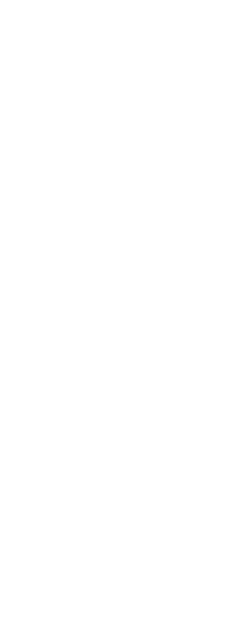Figure 4 shows the possibilities. A molecule in group (A) has its proton spins pointed in opposite directions, so that its total magnetic moment is zero. Chemists call this the para state. Molecules in groups (IB,), (C), and (D) are in what chemists call the ortho state. An ortho molecule has its proton spins pointing in the same direction, so that its total magnetic moment is 2.82 x 10-26 J/T. The three types of ortho molecules have their magnetic moments parallel (B), opposed (C), or in random directions perpendicular (D) to the external field. In normal circumstances, the number of molecules in each of the four groups-three ortho and one para - is roughly equal. All the magnetic moments cancel out, so that water normally has no net magnetic moment of its own. However, God was under no requirement to create the water molecules in their normal order. For example, He could have created all the molecules with their proton magnetic moments lined up in a given direction, producing the maximum magnetic moment possible from the protons. Or, He could have lined up the protons of the third ortho group (Figure 4(D)) along the field axis. Figure 5 shows this order. This would produce a field having one-fourth of the maximum strength with a minimum of deviation from the normal order. I do not know from Scripture what proportion of the protons God aligned in each case. In the previous article I put an arbitrary factor, k, into the equations. This alignment factor represents what fraction' of the maximum field God chose. The maximum value of k is one; the minimum is zero. Ordering by whole subgroups would give possible values of ¼, ½, ¾, or 1. In the previous paper I assumed that k for the earth was ¼. I supported this choice by pointing out that it increases the molecular order with a minimum of perturbation from the normal alignment. But it is a subjective choice. In the absence of any better criterion, let us assume that k = 0.25 unless we find out otherwise. Calculating the Fields Figure 3. Exponential decay curve, plotted on two types of graph. (a) Ordinary graph with linear (evenly-spaced) scales. Time T is the characteristic decay time. (b) Log-linear graph, having a linear horizontal scale and a logarithmic (compressed) vertical scale. An exponential decay is a straight line on such a graph. The magnetic fields of planets decay exponentially over thousands of years. The previous sections tell us everything we need to know to calculate a planet's magnetic moment at creation. It is simply the combined magnetic moment of all the aligned ortho water molecules.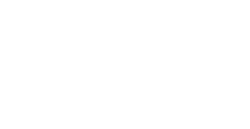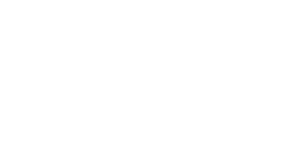Figure 4. Normal alignment of the hydrogen nuclei in water subjected to a weak magnetic field. Twenty-five percent of the water molecules are in each of the four possible states. Figure 5. One possible special alignment of water protons at creation. This configuration would produce 25 percent of the maximum possible magnetic field with not much deviation from the normal order.

The magnetic moment of mw of an ortho molecule is 2.82 x l0-26 J/T (section 3). The total number of water molecules comprising the planet at creation is the planet's mass m (in kg) divided by the mass mm of a water molecule, 2.992 x 10-26 kg. The factor k then gives us the fraction of aligned molecules. Putting all this together into an equation gives us the planet's magnetic moment M, at creation (in J/T):

Mc = k(m/mw)mw. (1)

This equation works out numerically as:

 Mc = k(0.9425 J/T kg )m. (2)

That is, every kilogram of water God created had a magnetic moment of nearly k joules per Tesla.

As I mentioned in the introduction, a large electric current would begin circulating in the planet's interior around the magnetic field axis, replacing the alignment of protons within seconds. The current would then decay exponentially.8 So the magnetic moment M at any time t after creation would be:

M = Mc exp(-t/T). (3)

Here T is the decay time, the time it takes for the field to decrease to 36.8 percent of any given value (Figure 3). Mc is the magnetic moment at creation given in equations (1) and (2). A planetary core of radius R (in meters) and uniform electrical conductivity s (in mhos per meter) would have a decay time of:9

T = (mosR2/p2) seconds, (4)

where mo, is the magnetic permeability (4p x 10-7 henry per meter).

Equations (1) or (2) can tell us the original magnetic moment of a planet. Measurements give us the present value. A straightforward reading of Scripture can give us the time t between creation and now. We can then solve equation (3) to give us the decay time of the planet's field:

T = t / ln(Mc/M). (5)

We can use this calculated decay time in equation (4) to calculate the average electrical conductivity of the planet's core:

s = p2T/moR2. (6)

If we have a reasonable estimate of the core radius, we can tell whether equation (6) gives us a reasonable value for the conductivity.

The Earth

Figure 6 shows how the Earth's measured dipole magnetic moment has decreased over the last 150 years.10, 11 The best fit to the data occurs with a decay time of:

T = 2049 + 79 years (data). (7)

The best-fit line gives a magnetic moment for the year 1980 A.D. of:

M = (7.94 + 0.05) x 1022 J/T (data). (8)

Table I contains a summary of the relevant solar system measurements.12 Using the table's value for the Earth's mass in equation (1) with k = 0.25 (section 3) gives my theory's estimate of the Earth's magnetic moment at creation:

Mc = 1.41 x 1024 J/T (theory). (9)

If the chronologic genealogies of Genesis 5 and 11 have no gaps in them, then the date of creation was about 4000 B.C.13 That would mean that the Earth in 1980 A.D. had an age of about 5980 years. Using these values in equation (5) gives this theory's estimate of the decay time of the Earth's magnetic field:

T = 2075 years (theory). (10)

This value agrees with the measured value in (7) to better than two percent, well within the experimental error. Using the experimental decay time (7) and the measured core radiusl4 of 3471 km in equation (6) gives us an estimate of the Earth's average core conductivity: 41900 + 1600 mhos/meter. This value is close to estimated conductivities of some materials under core conditions.15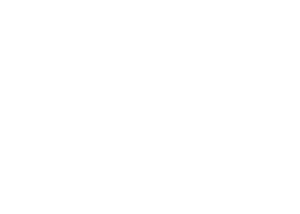The Sun The Sun has the largest magnetic moment of any object in the solar system. The fields at its surface are usually complex. They are very strong in some places, especially near sunspots. The Sun's magnetic fields and sunspot activity go through a fairly regular 22-year cycle16. When the number of sunspots is at a minimum, the Sun's general magnetic field is nearly dipolar.17 At that time, according to spectroscopic observations, the Sun's magnetic moment hag its maximum value:18-20 M ~ 3.5 x 1029 J/T (data). (11) Figure 6. Observed values of the Earth's magnetic dipole moment since 1829 A.D. A straight line on this log-linear graph implies an exponential decay. The straight line shown is the best (least-squares) fit to the data. The data are tabulated in reference I which come from references 10 and 11. This includes eight new points since Barnes' paper (Ref. 2). This value is only approximate because no space probe has orbited the Sun to make more accurate measurements. The magnetic moment does not stay at this peak level long. Over a period of years it steadily decreases to zero, reverses direction, and begins to increase again.

Eleven years after the first peak the dipole moment is again at a maximum, this time in the opposite direction. After another 11 years the dipole moment and direction are as they were at first. So the Sun's field reverses itself once every 11 years. If the solar cycle has stayed the same since creation, the field has reversed more than 500 times. If we now use the solar mass of Table I in equation (1) with k = 0.25, we get the following value for the solar magnetic moment at creation:

Mc = 4.65 x 1029 J/T (theory). (12)

This value is only about 25 percent higher than the latest observed peak. If this theory is correct, the Sun's magnetic field has not changed much since creation. The Sun's energies churn up the field, reversing it periodically, but they have not made the magnetic moment any larger. Instead, the churning seems to have decreased the field a bit. Using the values above in equation (5) gives an effective decay time of about 19000 years. I call this an "effective" decay time because it is shorter than the time we would calculate simply from equation (4). It means that the reversal cycles dissipate more energy than a simple decay does. This picture of the Sun's magnetic cycle differs from the one evolutionists imagine. They want to have solar and Planetary fields maintaining themselves by a dynamo (self-generating) process for billions of years.21

To accomplish this, the fluid motions in the Sun would have to crank as much energy into the magnetic field as various loss mechanisms dissipate. But no dynamo theory at present is mathematically specific enough to determine such quantities. In contrast to the dynamo theories, equation (12) implies that the field loses more than whatever it might gain, making the peak moment decrease a little each cycle. Figure 7 illustrates the difference. If the Sun is a dynamo, the engine does not seem to be chugging. The dynamo is running down.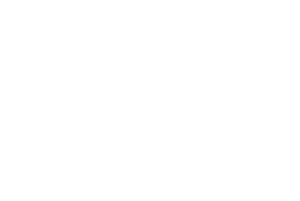The Moon Today the Moon has very little, if any, magnetic moment of its own. An analysis of the Apollo 15 lunar orbiter data sets the following upper limit on the Moon's present dipole moment:22 M < 1.3 x 1015 J/T (data). (13) But the Moon once had a strong magnetic field in the past. Lunar rock samples brought back by the Apollo crews had a natural remanent (permanent) magnetization acquired by cooling in an external magnetic field. Figure 7. The variation of the Sun's magnetic moment according to (a) dynamo theories, (b) this theory. If the peak magnetic moments are indeed decreasing, then the Sun does not have a self-generating dynamo. Some of the rocks were basalt, formed from cooling lava. Others were breccia, fragments probably pressed together by meteorite impacts. All magnetically tested samples had a remanent magnetization.23 But only a few were chemically stable enough to measure the

ancient field intensity by the most accurate method.24 The most accurately measured basalt is sample number 62235, taken by the Apollo 16 astronauts near the Descartes highlands.25 The basalt had been in a field of about 0.12 milliteslas (1.2 Gauss) when it cooled down. This intensity would be produced by a lunar magnetic moment of about:

Ml ~ 6.3 x 1021 J/T (data). (14)

The best-measured breccia is sample number 15498, taken by the Apollo 15 crew from Dune crater near the Lunar Appenines.26 It was in a 2100 nanotesla (0.021 Gauss) field during the meteorite impact. This corresponds to a lunar dipole moment of about:

M2 ~ 1.1 x 1020 J/T (data). (15)

I have assumed that these samples were near the magnetic equator when they cooled. If they were not, the dipole moments could be up to 50 percent smaller. Local irregularities in the ancient field could easily give a 50 percent error the other way, too. Most of the field intensities measured by the other (less accurate) methods give results between these two values. How do these values compare with the theory? Using the mass of the Moon (Table 1) and k = 0.25 in equation (1) gives us the magnetic moment of the Moon at creation:

Mc = 1.73 x 1022 J/T (theory). (16)

Using this value and the present value (13) in equation (5) shows that the decay time of the Moon's field is less than 364 years. This is a very short decay time. But it is a reasonable value, because the Moon has a very small core. Seismic measurements on the Moon give a core radius of about 350 km, which is consistent with other estimates.27 Using this radius and the above decay time in equation (6) shows that the Moon's average core conductivity is less than 30000 mhos/meter. This is about 75 percent of the value we got for the Earth's core. Such agreement is very close, considering the fact that small impurities can easily change the conductivity of a substance by an order of magnitude. It implies that the Earth and Moon could have similar core compositions.

We can now use the above decay time in equation (5) to estimate how long after creation the two lunar rocks were formed. Using the values (14) and (15) in turn for M in equation (5), and solving for t gives a formation time of less than 370 years after creation for the basalt and less than 1840 years for the breccia. Figure 8 shows the various times and dipole moments. The lava forming the basalt flowed less than a few centuries after creation. According to Genesis 5 and 7, the Flood occurred 1656 years after creation. So the meteorite forming the breccia hit the Moon less than a few centuries after the Genesis Flood.

Evolutionists have disagreed among themselves over how to solve what one called "the enigma of lunar magnetism."28 One faction points out that the data require a field source inside the Moon.29 They deduce from this that the Moon had a dynamo which later stopped. The other faction says that the Moon could-never have had a dynamo.30 First, its present slow rotation and small core are incompatible with most dynamo models. Second, current evolutionary theories cannot allow a molten core in the Moon's early history.Yet a molten core is essential to the dynamo theory. So this group keeps trying to find ways that some external field could have magnetized the Moon rocks. Both groups have started from a good point and then reached wrong conclusions. The Moon's field had an internal source, but it was not a dynamo. It was just an electric current running down. Here we have a simple creationist solution to a "paradox"31 that has puzzled evolutionists for a decade.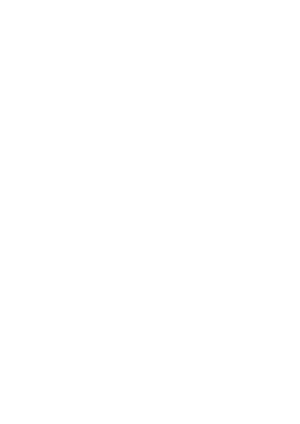The Inner Planets The Mariner 10 flybys of Mercury in 1974 and 1975 showed that the planet has a small but definite magnetic field. That surprised dynamo theorists, who had expected no field at all32 because of the planet's slow rotation. Mercury's lack of an atmosphere and an ionosphere make analysis of even a small field relatively simple. The magnetic dipole moment is:33 M = (4.8 0.3) x 1019 J/T (data). (17) Using k = 0.25 and Mercury's mass (Table 1) in equation (1) gives us its magnetic moment at creation: Mc = 7.5 x 1022 J/T (theory). (18) These two values, put into equation (5), give a decay time of about 813 years. From Mercury's density and other data, planetologists estimate that it has a fairly dense core with a radius of about 1830 km.14 Using this radius and this decay time in equation (6) gives us an average conductivity for Mercury's core of 60000 mhos/meter. This value is of the same order of magnitude as the core conductivities of the Earth and Moon. Mercury's magnetism is a problem for dynamo theorists: Figure 8. Decay of the Moon's magnetic moment. The present value is an upper limit. The initial value is from this theory. The values M1 and M2 are rough (factor of two) estimates of the lunar magnetic moment from the easured natural remanent magnetization of two lunar rocks (Ref. 23 and 25). The times for M1 and M2 are estimated from the decay curve. ... the very existence of the field is puzzling. If Mercury can maintain a steady dipole field, the earth, which rotates 59 times as fast and has a core twice as large, should be able to sustain more complicated fields.35 Again, this theory gives a simple answer to what seemed a difficult problem.

Measurements from both American and Russian space probes show small magnetic fields around Venus. Unlike Mercury, however, Venus has an atmosphere which complicates the analysis of small fields. At present, scientists feel that the planet's magnetosphere (ionosphere and solar wind) generates much of the observed field. If that is so, then the internally-generated magnetic moment of Venus is less than:36, 37

M < 1019 J/T (data). (19)

Using k = 0.25 and the planet's mass (Table 1) in equation (1) gives us the dipole moment of Venus at creation:

Mc = 1.15 x 1024 J/T (theory). (20)

This implies, by equation (5), a decay time of less than 513 years. If the core radius is about 2700 km,38 this rapid decay implies from equation (6) that the core conductivity of Venus is less than 17000 mhos/meter.

Dynamo theorists say that the slow rotation of Venus explains its low field. But our creationist theory offers an explanation which is at least as good: that the core of Venus is less than half as conductive as the Earth's. The various space missions to Mars have shown that it, like Venus, has a small magnetic field. The upper limit to its dipole moment is:39

M < 2.1 x 1011 J/T (data). (21)

Again, using k = 0.25 in equation (1) gives the dipole moment of Mars at creation:

Mc = 1.51 x 1023 J/T (theory). (22)

These values imply a decay time of less than 535 years. Using an estimated core radius of 1750 km shows that Mars' core conductivity is less than 43000 mhos/meter.

This is about the same as the Earth's conductivity. So for the Moon and the terrestrial planets, we find similar core conductivities. But the dynamo theories do not produce such consistency:

Mars has no field, although it rotates more than 50 times as fast as Mercury. If Mars' core is comparable in size to Mercury's, as some workers have argued on the basis of the mean density of the planet, the absence of a field on Mars and the presence of one on Mercury is baffling.40

Table I

Solar System Physical Data

From Reference 12

 No. Body Mass (kg) Avg. Radius (km) Avg. Density (g/cm3) Rotation Period (days) 1. Sun 1.991 x 1030 695,950 1.410 24.66 2. Mercury 3.181 x 1023 2,433 5.431 58.82 3. Venus 4.883 x 1024 6,053 5.256 244.59 4. Earth 5.979 x 1024 6,371 5.519 1.00 5. Moon 7.354 x 1022 1,738 3.342 27.40 6. Mars 6.418 x 1023 3,380 3.907 1.03 7. Jupiter 1.901 x 1027 69,758 1.337 0.41 8. Saturn 5.684 x 1026 58,219 0.688 0.43 9 Uranus 8.682 x 1025 23,470 1.603 0.45 10. Neptune 1.027 x 1026 22,716 2,272 0.66 11. Pluto (1.08 1.00) x 1024 5,700 1.65 1.57 6.41

In summary, the creation/decay theory explains the magnetism of the inner planets more consistently than the dynamo theory does.

The Outer Planets

Jupiter is the largest and most massive object orbiting the Sun. The Pioneer and Voyager missions to this awesome planet showed that it also has an intense magnetic field. Its magnetic moment is second only to the Sun's:41

M = 1.55 x 1027 J/T (data). (23)

If we use our arbitrary value of k = 0.25 in equation (1) to calculate Jupiter's magnetic moment at creation, we get a value less than this. The minimum alignment fraction which will give the present field is 0.87. But since the field must have decayed at least somewhat since creation, the fraction must have been greater. If we use the maximum alignment fraction, k = 1.0, then we get a maximum value for Jupiter's magnetic moment at creation:

Mc < 1.79 x 1027 J/T (theory). (24)

So it looks as if God pulled out nearly all the organ stops when He orchestrated Jupiter. Not only did He create a larger mass of water, but He lined up more than 90 percent of the water's hydrogen nuclei. These two values imply that Jupiter's decay time is greater than 41000 years.

We have no direct measurements on Jupiter's core radius R yet. But we can use Jupiter's average surface radius Rs, 70000 km, to express the average core conductivity of equation (6) as a function of R/Rs:

s = (p2T/mo R2s)(Rs/R)2. (25)

Using the above decay time in (25) gives us a minimum value for Jupiter's core conductivity:

s > (Rs/R)2(2100 mhos/meter). (26)

From Jupiter's low density and equatorial bulge, planetologists estimate that it is mostly hydrogen and some helium with a rocklike inner core. The hydrogen is probably in a liquid state (possibly metallic also) below about 0.8 Jovian radii from the center.42 Using this in equation (26) shows that the average core conductivity is greater than 3000 mhos/meter. This value is some what lower than theoretical estimates of the conductivity of liquid metallic hydrogen. But it is consistent with the estimated conductivity of liquid molecular hydrogen.43 At present there are not enough experimental data on the conductivity of hydrogen at high pressures and temperatures to shed further light.

Saturn is also a low-density planet like Jupiter, but somewhat smaller. Pioneer 11 measurements show that its magnetic moments:44, 41

M = 4.3 x 1025 J/T (data). (27)

Using k = 0.25 and Saturn's mass from Table 1 gives its magnetic moment at creation:

Mc = 1.34 x 1026 J/T (theory). (28)

These two values give a decay time of 5300 years. Using an average surface radius of 58000 km in equation (25) gives Saturn's average core conductivity:

s = (Rs/R)2(390 mhos/meter). (29)

If the core radius is about 0.6 Saturn radii,46 its average conductivity is about 1100 mhos/meter. So the two gas giant planets have similar core conductivities according to this theory.

Until Voyager 2 flies past Uranus in January 1986, we will not have any direct measurements of that planet's magnetic field. However, observations of ultraviolet light from atomic hydrogen in the atmosphere of Uranus provide good indirect evidence that the planet does have a field.47 Using the mass from Table I and assuming that k = 0.25 gives Uranus' magnetic moment at creation:

Mc = 2.05 x 1021 J/T (theory). (30)

The maximum value (for k = 1.0) according to this theory would be 8.18 x 1025 J/T. One recent speculative model of Uranus has a dense core of about 8000 km radius surrounded by an icy mantle.48 If the core conductivity is similar to that of the inner planets, it would be of the order of 104 mhos/meter. In that case, according to equation (3), (4), and (30), the present dipole moment of Uranus would be of the order of 1024 J/T.

Voyager 2 may go on to a rendezvous with Neptune, the eighth planet, in late 1989. If it is successful, it will provide the only measurements to date on Neptune's magnetic field. Using the Table I value for Neptune's mass and k = 0.25 gives an estimate of Neptune's magnetic moment at creation:

Mc = 2.42 x 1025 J/T (theory). (31)

The maximum value (for k = 1.0) would be 9.68 x 1021 J/T. Neptune is supposed to have a core and icy mantle similar to those of Uranus.49 If that model is correct, we would expect Neptune to have a similar present magnetic moment, of the order of 1024 J/T.

If Neptune does have a field, it would make some difficulties for the dynamo theorists, because its core is supposed to be solid. A solid conductive core is no hindrance to the creationist theory, but it is to the dynamo theory.

Voyager 2 is not scheduled to visit Pluto, the ninth planet. We do not even know its mass very well. From the Table I value and k = 0.25, we estimate its magnetic moment at creation as:

Mc = (2.55 + 2.37) x 1024 J/T (theory). (32)

Pluto's density indicates that it is mostly ice, which has a low conductivity. If that is so, we would not expect Pluto to have any appreciable magnetic moment at present.

In summary, the magnetism of the outer planets falls within the bounds of our creationist theory. Jupiter's huge field is close to, but under, the limit set by using k = 1.0 in the theory. Saturn's field is more typical. The theory indicates that both planets have similar core conductivities, which is consistent with their similar structure. The upcoming Voyager 2 visits to Uranus and Neptune offer a chance to further test the creationist and evolutionist theories. If either of the two planets has a field stronger than the k = 1.0 limit, it would be evidence against the creationist theory. On the other hand, if either planet lacks a conductive fluid interior and yet has a sizable field, it would weigh heavily against the dynamo theory.

Conclusions

Table 11 and Figure 9 summarize the magnetic field data. The magnetic dipole moments cover a very wide range, from 1015 J/T to 1030 J/T. All the data fall within the bounds of the theory I am presenting. No solar system body yet measured has a magnetic moment greater than the k = 1.0 limit. Yet all of the bodies show evidence of having once had a magnetic moment a sizable fraction of that limit.

Evolutionists often say that creationist theories are not "real science" because, they claim, such theories make no predictions which can be tested. But in this theory we have a counterexample to their claim. Here are some specific predictions of the theory which could be tested by future data from space missions:

1. Older igneous rocks from Mercury or Mars should have natural remanent magnetization, as the Moon's rocks do.

2. Mercury's decay rate is so rapid that some future probe could detect it fairly soon. In 1990 the planet's magnetic moment should be 1.8 percent smaller than its 1975 value.

3. The upcoming Voyager 2 encounters with Uranus and Neptune should show planetary magnetic moments less than the k = 1.0 limit: 8.2 x 1015 J/T for Uranus and 9.7 x 1025 J/T for Neptune.

Table II

Solar System Magnetic Data

 No. Body Magnetic Moment at Creation (J/T) Present Magnetic Moment (J/T) Decay Time (years) Core Radius (km) Core Conductivity (mho/meter) 1. Sun 4.7 x 1029 3.5 x 1029 19,000 2. Mercury 7.5 x 1022 4.8 x 1019 810 1800 60,000 3. Venus 1.2 x 1024 <1.0 x 1019 <510 2700 <17,000 4. Earth 1.4 x 1024 7.9 x 1022 2075 3480 42,000 5. Moon 1.7 x 1022 <1.3 x 1015 <360 350 <30,000 6. Mars 1.5 x 1023 < 2.1 x 1018 <540 1750 <43,000 7. Jupiter 1.8 x 1027 1.6 x 1027 >41,000 56,000 >3,000 8. Saturn 1.3 x 1026 4.3 x 1025 5,300 35,000 >1,100 9. Uranus 2.1 x 1025 10. Neptune 2.4 x 1025 11. Pluto 2.6 x 1024

Magnetic moments at creation are from this theory with k = 0.25, except for the case of Jupiter, where k = 1.0. Present magnetic moments are measured values. D2cay times are deduced front created and present moments. Core radii of the Earth and Moon are measured; all others are estimates from current planetary models. Core electrical conductivities are deduced from other items. The deduced decay time for the Earth agrees well with the observed decay time of 2049+79 years.

There are several important points I want to emphasize:

Magnetic reversals are not conclusive proof of a self generating dynamo. In section 6 we saw that though the Sun's energies reverse its field periodically, the present field is no stronger than the created field. Applying this to the Earth's field means that evidence for possible reversals in the past does not contradict the creation-decay theory. The Earth's field could, for example, have decayed steadily from creation to the Flood, reversed rapidly many times during the upheavals of the Flood, and afterwards resumed its steady decay.

This theory is more comprehensive than the dynamo theories. As far as I can tell, it explains everything the dynamo idea does, but in a simpler, more quantitative way. It also explains the presence of a field on Mercury, the absence of one on Mars, and the former field of the Moon - all of which puzzle evolutionists.

The solar system is young. It would be impossible to understand the field strengths of the Earth, Moon, and inner planets on the basis of this theory without a time scale of roughly 6000 years.

Water was the raw material of creation. This theory would not work using the present composition of the solar system. The nuclei of the Moon and inner planets have too little magnetic moment. The hydrogen nuclei of the Sun and outer planets have too much. Only the proportion of hydrogen in an equal mass of water gives the right results.

The Bible is scientifically accurate. A straightforward reading of Scripture supplied the essentials of this theory: the possibility of initial alignment, the water composition, and the short time scale. The fact that the theory fits the facts shows that the scientist can rely on the Bible for new insight into the natural world.

Finally, notice that we find magnetic fields of the right magnitudes throughout the solar system. This may be true throughout the whole universe. I have done "back of the envelope" calculations for white dwarf stars, which have the strongest magnetic fields yet observed in nature. Although the subject of stellar magnetic fields belongs properly to another paper, the theory appears to fit them, too.

In this light we see that what one evolutionist called "the ubiquity of magnetic fields" in the cosmos50 is really a clue to creation. It is the Creator's signature upon his artistry.

"The heavens declare the glory of God, And the expanse shows forth His handiwork." Psalm 19:1.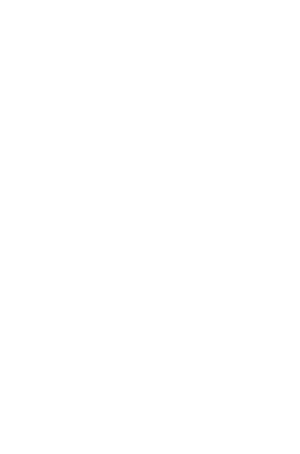References 1. Humphreys, D. R. 1983. The creation of the Earth's magnetic field. Creation Research Society Quarterly, 20(2): 89-94. 2. Barnes, T. G. 1973. Electromagnetics of the Earth's field and evaluation of electric conductivity, current, and joule heating in the Earth's core. Creation Research Society Quarterly, 9(4):222-230. 3. Allen, C. W. 1976. Astrophysical quantities. Athalone Press, London. Third edition, p. 27. 4. Stacey, F. D. 1969. Physics of the Earth. John Wiley & Sons, New York, p. 276. 5. Humphreys, D. R. 1978. Is the Earth's core water? Part one: the Biblical evidence. Creation Research Society Quarterly, 15(3):141-147. See reference 9, p. 146. The word translated "heavens" is shamayim. The first syllable, sham, means "there." The second syllable mayim, means "waters." See Holladay, W. L. 1971. A concise Hebrew and Aramaic lexicon of the Old Testament. W. B. Eerdmans Co., Grand Rapids, Michigan, pp. 374, 375, 193. Figure 9. Magnetic moments in the solar system since creation. The initial values come from this theory. The present values are from experimental measurements. Triangles represent upper limits. 6. Evans, R. D. 1955. The atomic nucleus. McGraw-Hill Book Co., New York, pp. 152-155. 1 am using the value 2.793 nuclear magnetons for the proton magnetic moment. A nuclear magneton is 5.050 x 10-27 I/T.

7. Davis, J. C. 1965. Advanced physical chemistry. Ronald Press Co., New York, p. 297.

8. Barnes. Op. cit.

9. Barnes. Op. cit.

10. McDonald, K. L. and R. H. Gunst. 1967. An analysis of the Earth's magnetic field from 1835 to 1965. Environmental Services Administration Technical Report IER 46- IESI, U.S. Dept. of Commerce, p. 15.

11. Langel, R., R. Estes, G. Mead, E. Fabiano, and E. Lancaster. 1980. Initial geomagnetic field model from magsat vector data. Geophysical Research Letters, 7(10):793-796. (Multiply values in Table 5 by 2.586 x 1018 to get M in J/T.)

12. Weast, R. C., editor. 1982. CRC handbook of chemistry and physics. CRC Press, Boca Raton, Florida, 62nd edition, pp. F-140, F-142

13. Niesson, R. 1982. A Biblical approach to dating the Earth: a case for the use of Genesis 5 and 11 as an exact chronology. Creation Research Society Quarterly, 19(l):60-66.

14. Allen. Op. cit., pp. 117-118.

15. Stacey, F. D. 1967. Electrical resistivity of the Earth's core. Earth and Planetary Science Letters, 3:204-206. Sta cey estimates the conductivity of a nickel-iron-silicon mixture as of the order of 30000 mhos/meter. He points out that other light elements, such as magnesium or oxygen, would have the same effect as silicon.

16. Newkirk, G., and K. Frazier. 1982. The solar cycle. Physics Today, 35(4):25-34.

17. Sheely, N. R. 1981. The influence of differential rotation on the equatorial component of the Sun's magnetic field. Astrophysical Journal, 243:1040-1048 (February 1).

18. Akasofu, S., P. Gray, and L. Lee. 1980. A model of the heliospheric magnetic field configuration. Planetary Space Science, 28:609-615. During his visit to Sandia National Laboratories on October 5, 1983, Dr. Akasofu told me that his value is based on an approximate value of two gauss for the general field near the Sun's poles at spot minimum taken from Allen's book (Ref. 3, p. 161) and elsewhere. Allen actually says "1 or 2 gauss." References 19 and 20 below are more detailed studies giving the same, result.

19. Howard, R. 1977. Studies of solar magnetic fields; V: The true average field strengths near the poles. Solar Physics, 52:243-248.

20. Svalgaard, L., T. Duvall, and P. Scherrer. 1978. The strength of the Sun's polar fields. Solar Physics, 58:225-240.

21. Newkirk, Op. cit., p. 27.

22. Russell, C., P. Coleman, and G. Schubert. 1974. Lunar magnetic field: permanent and induced dipole moments. Science, 186:825-826.

23. Gose, W., D. Strangway, and G. Pearce. 1973. A determination of the intensity of the ancient lunar magnetic field. The Moon, 7:196-201.

24. Dunlop, D., M. Bailey, and M. Westcott-Lewis. 1975. Lunar paleointensity determination using anhysteretic remanence (ARM): a critique. Proceedings of the Lunar Science Conference, 6th, pp. 3063-3069.

25. Stephenson, A., K. Runcorn, and D. Collinson. 1975. On changes in the intensity of the ancient lunar magnetic field. Proceedings of the Lunar Science Conference, 6th, pp. 3049-3062.

26. Gose. Op. cit.

27. Runcorn, S. K. 1983. Lunar magnetism, polar displacements and primeval satellites in the Earth-Moon system. Nature, 304:589-596. See p. 591 for lunar core size review.

28. Hood, L. L. 1981. The enigma of lunar magnetism. Eos, 62(16):161-163.

29. Runcorn. Op. cit.

30. Hood. Op. cit.

31. Driscoll E, 1972. The magnetic moon: How did it get that way? Science News, 101(22):346-347 (May 27). Driscoll quotes Paul W. Gast of NASA's Manned Spacecraft Center as saying: "It would be much simpler to explain most of the things we understand about the moon, if we could somehow dispose of this magnetic field.... One of the exciting things about this paradox or enigma is [that] perhaps behind all of this is an explanation that none of us are thinking about today."

32. Parker, E. N. 1983. Magnetic fields in the cosmos. Scientific American, 249(2):44-54 (August). See p. 51.

33. Ness, N. F. 1979. The magnetic field of Mercury. Physics of the Earth and Planetary Interiors, 20:209-217.

34. Ness. Op. cit., p. 215, using a core radius 0.75 of the planet's radius.

35. Parker. Op. cit., p. 52.

36. Russell, C., R. Elphic, and J. Slavin. 1979. Initial Pioneer Venus magnetic field results: nightside observations. Science, 205:114-116 (July 6).

37. Dolginov, S., et al. 1981. Field configuration in the magnetic tail of Venus. Cosmic Research, 19(4):434-442 (July-August).

38. Bullen, K. E. 1973. Cores of the terrestrial planets. Nature, 243:68-70 (May 11). Based on an iron oxide core model.

39. Russell, C. T. 1979. The Martian magnetic field. Physics of the Earth and Planetary Interiors, 20:237-246.

40. Parker. Op. cit., p. 52.

41. Connerney, J., M. Acuna, and N. Ness. 1982. Voyager I assessment of Jupiter's planetary magnetic field. Journal of Geophysical Research, 86(A5):3623-3627 (May 1). On p. 3625 the authors list the spherical harmonic coefficients of the field. To get the dipole moment in gauSS-CM3, Multiply the cube of the planet's radius (in cm) by the square root of the sum of the squares of the first three coefficients. Divide the result by 1000 to get the moment in J/T.

42. Ingersoll, A. 1981. Jupiter and Saturn. Chapter 12 of The New Solar System, edited by J. Beatty, B. O'Leary, and A. Chaikin. Cambridge University Press, New York, pp. 117-128.

43. Smoluchowski, R. 1975. Jupiter's molecular hydrogen layer and the magnetic field. Astrophysical Journal, 200:L119-LI21 (Sept. 1).

44. Acuna, M., N. Ness, and J. Connerney. 1980. The magnetic field of Saturn: further studies of the Pioneer 11 observations. Journal of Geophysical Research, 85(All):5675-5678 (Nov. 1).

45. Acuna, M., J. Connerney, and N. Ness. 1983. The z zonal harmonic model of Saturn's magnetic field: analyses and implications. Journal of Geophysical Research, 88(All): 8771-8778 (Nov. 1).

46. Hughes, D. W. 1983. Inside the giant planets. Nature, 305:669-670 (Oct. 20).

47. Durrance, S. T. and H. W. Moos. 1982. Intense L emission from Uranus. Nature, 299:428-429 (Sept. 30).

48. Smoluchowski, R. 1983. The interiors of the giant planets-1983. The Moon and the Planets, 28:137,154.

49. Ibid.

50. Parker. Op. cit., p. 45.